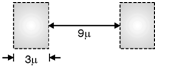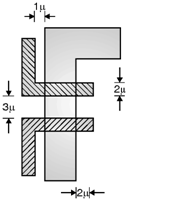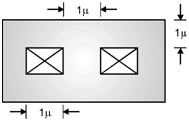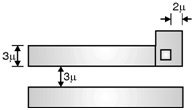Home > Digital CMOS Design > CMOS Layout Design > Micron Design Rules

Micron-Design-Rules

Micron () Design Rules : Industry uses the micron design rules and code designs in terms of these micron dimensions. The micron design rules are as follows :

(1) Rules for N-well as shown in Figure below.
1. Width = 3
2. Space = 92) Rules for active area as shown in Figure below.
1. Minimum size = 3
2. Minimum spacing = 3
2. N+ active to N-well = 7(3) Rules for poly 1 as shown in Figure below.
1. Width = 2
2. Spacing = 3
3. Gate overlap of active = 2
4. Field poly 1 to active = 1(4) Rules for contact to poly 1 as shown in Figure below.
1. Exact contact size = 2  2
2. Minimum poly overlap = 1
3. Minimum contact spacing = 2(5) Rules for contact to active as shown in Figure below.
1. Exact contact size = 2  2
2. Minimum active overlap = 1
3. Minimum contact spacing = 2
4. Minimum spacing to gate = 2(6) Rules for metal 1 as shown in Figure below.
1. Width = 3
2. Spacing = 3
3. Overlap of contact = 1
4. Overlap of via = 2(7) Rules for metal 2 as shown in Figure below.
1. Width = 3
2. Space = 3
3. Metal 2 overlap of via = 2